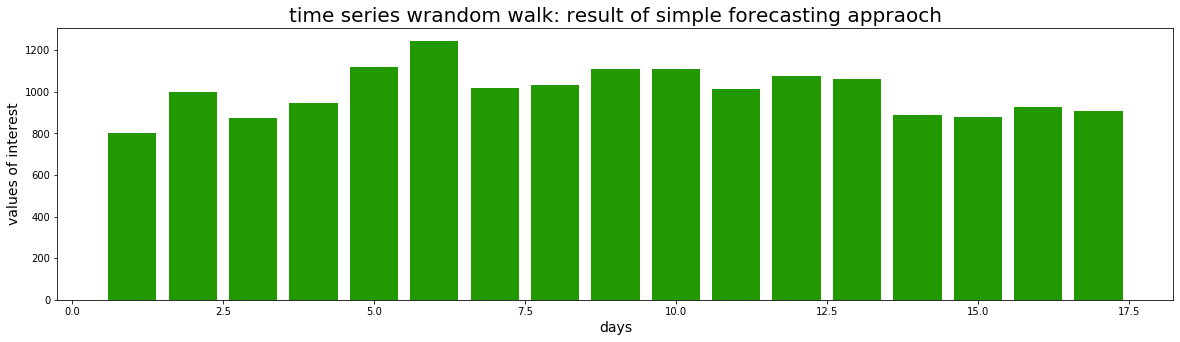# Implementing a random walk forecast in PythonIn previous posts I introduced very simple (and naive) forecasting methods, namely CAGR-based forecasting and simple moving average forecasting. I implemented such forecasting methods in R and demonstrated basic use cases.

In this post I want to introduce another simple forecasting method: Random walk forecasting. I will implement an example using Python.

Random walk forecasting can e.g. be used for creating a simple baseline model against which other forecasts can be compared. A random walk can be a good benchmark. Here I am e.g. thinking of financial market analysis where many financial engineers try to create trading strategies and forecasting models that can be tested against random walks.

Below I implement a random walk forecasting function in Python. The function requires the random module.

```import random
# function for simulating a random walk; parameters include annual growth rate, starting point etc.
def random_walk_forecast(annualGrowth,
startPoint,
forecastLength,
productiveDaysPerYear,
standardDeviationRelativeToMeanGrowth):
# declare empty prediction return list
prediction = []
# convert annual growth into daily growth, assuming defined number of productive days per year
g = (1+annualGrowth)**(1/productiveDaysPerYear)-1
# calculate all prediction values
for i in range(0,forecastLength):
# if first prediction then list is still empty
if i == 0:
prediction.append(startPoint*random.normalvariate(mu=1+g,
sigma=g*standardDeviationRelativeToMeanGrowth))
else:
prediction.append(prediction[i-1]*random.normalvariate(mu=1+g,
sigma=g*standardDeviationRelativeToMeanGrowth))
# return the list of predicted values
return prediction
```

Using the random_walk_forecast we can visualize a random walk forecast using pyplot, as part of the matplotlib library in Python:

```# list with historical data
historicals = [800,1000,874,945,1121,1245,1020]
# assumed annual growth rate
growth_rate = 0.03
# prediction, assuming 220 productive days per year; forecasting for 10 work day horizon
predictions = random_walk_forecast(annualGrowth = growth_rate,
startPoint = historicals[-1],
forecastLength=10,
productiveDaysPerYear=220,
standardDeviationRelativeToMeanGrowth = 500)
# import matplotlib.pyplot for being able to create plots
import matplotlib.pyplot as plt
# set figure size
plt.figure(figsize = (20,5))
# merge historicals and predictions; lets extend predictions onto historicals
historicals.extend(predictions)
# create a simple bar plot
plt.bar(range(1,len(historicals)+1),
historicals,
color = "#229900")
# add a title to the graph
plt.title("time series wrandom walk: result of simple forecasting appraoch",size=20)